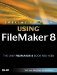# Using Conditional Functions

Conditional functions are used when you want to return a different result based on certain conditions. The most basic and essential conditional function is the If() function. If takes three parameters: a test, a true result, and a false result. The test needs to be a full equation or expression that can be evaluated as true or false.

Let's look at an example. Suppose that you have a set of records containing data about invoices. You'd like to display the status of the invoice"Paid" or "Not Paid"based on whether the AmountDue field has a value greater than zero. To do this, you'd define a new field, called InvoiceStatus, with the following formula:

`If ( AmountDue > 0, "Not Paid", "Paid")`

For each record in the database, the contents of the InvoiceStatus field will be derived based on the contents of that record's AmountDue field.

The test can be a simple equation, as in the preceding example, or it can be a complex test that uses several equations tied together with and and or logic. For the test

`If ( A and B; "something"; "something else")`

both A and B have to be true to return the true result. However, for the test

`If ( A or B; "something"; "something else" )`

if either A or B is true, it will return the true result.

The true or false result arguments can themselves be If() statements, resulting in what's known as a nested If() statement. This allows you to test multiple conditions and return more than two results. For instance, let's revise the logic of the InvoiceStatus field. Say that we wanted invoices with a negative AmountDue to evaluate as Credit Due. We could then use the following field definition:

`If ( Amount Due > 0; "Not Paid"; If (Amount Due < 0; "Credit Due"; "Paid" ))`

The other commonly used conditional function is the Case () statement. The Case () statement differs from the If () statement in that you can test for multiple conditions without resorting to nesting. For instance, say that you have a field called GenderCode in a table that contains either M or F for a given record. If you wanted to define a field that would display the full gender, you could use the following formula:

`Case ( GenderCode = "M"; "Male"; GenderCode="F"; "Female" )`

A Case () statement consists of a series of tests and results. The tests are conducted in the order in which they appear. If a test is true, the following result is returned; if not, the next test is evaluated. FileMaker stops evaluating tests after the first true one is discovered. You can include a final optional result that is returned if none of the tests comes back as true. The gender display formula could be altered to include a default response as shown here:

`Case ( GenderCode = "M"; "Male"; GenderCode="F"; "Female"; "Gender Unknown" )`

Without the default response, if none of the tests is true then the Case () statement returns a null value.Special Edition Using FileMaker 8
ISBN: 0789735121
EAN: 2147483647
Year: 2007
Pages: 296